# abacus

(redirected from Abacus arithmetic)
Also found in: Dictionary, Thesaurus, Financial.

## abacus

### Bibliography

See J. M. Pullan, The History of the Abacus (1968); P. H. Moon, The Abacus (1971).

## abacus

(ăb`əkəs), in architecture, flat slab forming the top member of a capital. In classical orders it varies from a square form having unmolded sides in the Greek Doric, to thinner proportions and ovolo molding in the Greek Ionic, and to sides incurving and corners cut in Roman Ionic and Corinthian examples. In Romanesque work the abacus is heavier in proportion, projects less, and is generally molded and decorated. In Gothic work the form varies, appearing in square, circular, and octagonal forms with molded members.

## Abacus

The flat area at the top of a capital, dividing a column from its entablature. It usually consists of a square block, or is enriched with moldings. In some orders the sides are hollowed and the angles at the corner are truncated.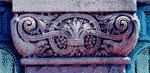## Abacus

a device used to carry out arithmetic calculations (Figure 1). Although more advanced calculating machines are now in use, the abacus is still employed in many countries for practical computations.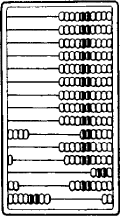Figure 1. Russian abacus; the number represented is 401.28

The forerunner of the modern Russian abacus was the doshchanyi schet (counting board), which first appeared in Russia in the 16th century. The development of the doshchanyi schet was greatly influenced by the system of taxation used in Russia from the 15th to 17th centuries (seeSOSHNOE PIS’MO). This system required the addition, subtraction, multiplication, and division not only of whole numbers but also of fractions, since the unit of taxation, the sokha, was divisible into parts.

The doshchanyi schet consisted of two frames that could be folded together. Each frame was divided into two sections (in later types only the lower part of the frame was so divided). The second frame was needed because of the nature of the monetary system. Counters were strung on taut strings or wires running across the frame. Since the decimal number system was used, each whole-number row had nine or ten counters (Figure 2). Operations on fractions were performed on incomplete rows: a row of three counters represented three thirds, and a row of four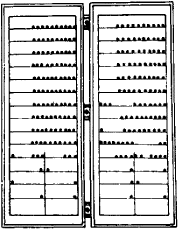Figure 2.

Note on Figure 2.Doshchanyi schet (from a 17th century drawing); the number represented on the left is ¼ + ⅙ +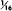, and the amount shown on the right is 30 rubles 18 altynyden’gi

counters represented four fourths. Located under these fraction rows were rows containing one counter each: each counter represented one-half of the fraction it was under. For example, the counter under the row with three counters represented one-half of one-third, and the counter under this counter represented one-half of one-half of one-third. Fractions were added without reduction to a common denominator; an example of a typical sum is 1/4 + 1/6 + 1/16. Sometimes operations on fractions were performed as operations on whole numbers by setting the sokha equal to a certain amount of money. If, for example, the sokha is equal to 48 monetary units, then the above fraction is equal to 12 + 8 + 3 = 23 monetary units.

The introduction of Arabic numerals and a new taxation system entailed the loss of the rows for fractions in the late 17th century. In the early 18th century, the second frame was eliminated, and the Russian abacus acquired its present appearance. A single incomplete row was retained. Usually containing four counters, it separates the two rows used for tenths and hundredths from the other rows and is sometimes used for fourths and halves. Outside the USSR, the Russian abacus is used in Iran. Abaci developed in the 19th century on the basis of the Russian abacus are employed as teaching aids in Western Europe.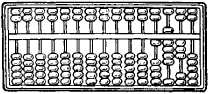Figure 3. Chinese abacus; the number represented is 1,930

Considerably older than the Russian abacus is the Chinese abacus, or suan-pan (Figure 3), which still retains its ancient structure. Units are counted up to five, and then fives are counted. Versions of the Chinese abacus are used in Indochina and Japan.

### REFERENCE

Spasskii, I. G. “Proiskhozhdenie i istoriia russkikh schetov.” In Istoriko-matematicheskie issledovaniia, fasc. 5. Moscow, 1952.

## abacus

[′ab·ə‚kəs]
(architecture)
A slab forming the topmost division of the capital of a column.
(mathematics)
An instrument for performing arithmetical calculations manually by sliding markers on rods or in grooves.

## abacus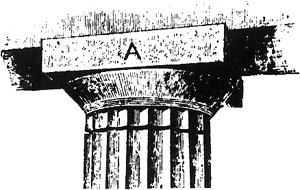abacus A
The uppermost member of the capital of a column; often a plain square slab, but sometimes molded or otherwise enriched.

## abacus

1. a counting device that consists of a frame holding rods on which a specific number of beads are free to move. Each rod designates a given denomination, such as units, tens, hundreds, etc., in the decimal system, and each bead represents a digit or a specific number of digits
2. Architect the flat upper part of the capital of a column

## abacus

One of the earliest counting instruments. Similar devices predate the Greek and Roman days. It uses sliding beads in columns that are divided in two by a center bar. The top is "heaven," where each of two beads is worth 5 when moved to the center bar. The bottom is "earth," where each of five beads is worth 1 when moved toward the center. See biquinary code.

An Abacus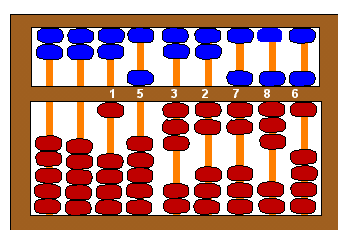The number appearing on this Chinese abacus is 1,532,786.
Site: Follow: Share:
Open / Close